Normal view MARC view ISBD view

# Probability for statistics and machine learning : fundamentals and advanced topics / Anirban DasGupta.

Material type:TextSeries: Springer texts in statistics.Publisher: New York : Springer, c2011Description: xix, 782 p. : ill. ; 24 cm.ISBN: 1441996338; 9781441996336; 9781441996343 (ebk.).DDC classification: 519.2 Online resources: WorldCat details | E-book Fulltext
Contents:
Table of contents Review of univariate probability -- Multivariate discrete distributions -- Multidimensional densities -- Advanced distribution theory -- Multivariate normal and related distributions -- Finite sample theory of order statistics and extremes -- Essential asymptotics and applications -- Characteristics functions and applications -- Asymptotoics of extremes and order statistics -- Markov chains and application -- Random walks -- Brownian motion and Gaussian processes -- Poisson processes and applications -- Discrete time martingales and concentration inequalities -- Probability metrics -- Empirical processes and VC theory -- Large deviations -- The exponential family and statistical applications -- Simulation and Markov chain Monte Carlo -- Useful tools for statistics and machine learning.
Summary: Summary: This accessible book provides a versatile treatment of classic as well as modern probability theory, while integrating them with core topics in statistical theory and also some key tools in machine Read more...
Tags from this library: No tags from this library for this title.Average rating: 0.0 (0 votes)
Item type Current location Collection Call number Copy number Status Date due Barcode Item holdsE-Book
E-book
Non-fiction 519.2 DAP 2011 (Browse shelf) Not for loanText
Reserve Section
Non-fiction 519.2 DAP 2011 (Browse shelf) C-1 Not For Loan 26617
Total holds: 0
##### Browsing EWU Library Shelves , Shelving location: E-book Close shelf browser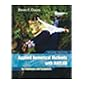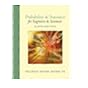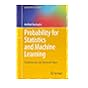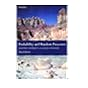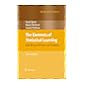518 CHA 2008 Applied numerical methods with MATLAB for engineers and scientists / 518 YAA 2005 Applied numerical methods using MATLAB / 519.02462 PRO 2014 Probability and statistics for engineers and scientists / 519.2 DAP 2011 Probability for statistics and machine learning : 519.2 GRP 2001 Probability and random processes / 519.2 HAE 2009 The elements of statistical learning : 519.2 MEI 2013 Introduction to probability and statistics /

Includes bibliographical references and indexes.

Multivariate discrete distributions --
Multidimensional densities --
Multivariate normal and related distributions --
Finite sample theory of order statistics and extremes --
Essential asymptotics and applications --
Characteristics functions and applications --
Asymptotoics of extremes and order statistics --
Markov chains and application --
Random walks --
Brownian motion and Gaussian processes --
Poisson processes and applications --
Discrete time martingales and concentration inequalities --
Probability metrics --
Empirical processes and VC theory --
Large deviations --
The exponential family and statistical applications --
Simulation and Markov chain Monte Carlo --
Useful tools for statistics and machine learning.

Summary:
This accessible book provides a versatile treatment of classic as well as modern probability theory, while integrating them with core topics in statistical theory and also some key tools in machine Read more...

Applied Statistics

There are no comments for this item.